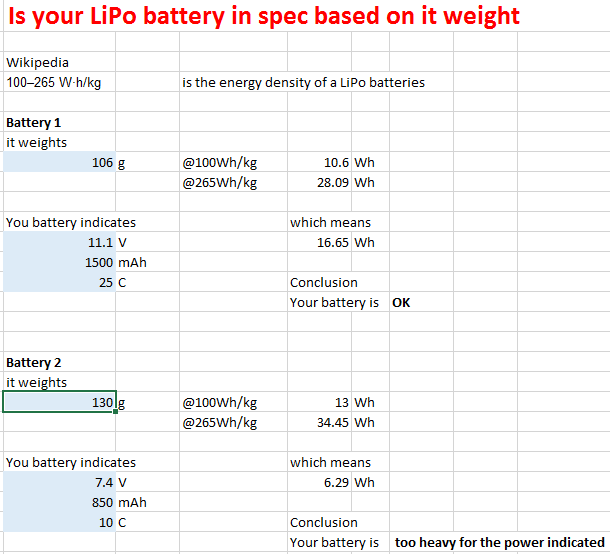## About Batteries, their weight, capacity, etc...

I ordered some batteries online at Banggood and they were all 3S batteries, meaning 11.1V, 1500mAh.

I was surprise to see how different they looked. The main difference was the weight. The heaviest was 136grams and the lightest was just 106grams.

Because energy in a battery is proportional to the volume, how can you have the same capacity of 1500mAh and 11.1V if you have such a different weight.

So I looked on Wikipedia, and found that the LiPo technology has an energy density of 100 to 265Wh/kg  which make a ratio of 265% difference from one battery to the other.

My conclusion here was that in that case, this difference of 35% in weight was still less than the 265% in energy density so I decided to see how they behave in real life.

I made a small Excel Template to calculate if the batteries I am buying are in spec or ou of specification in regards to the weight they have.All it does is to calculate the Energy your battery can hold by multiplying the amperage (i.e. 1500mAh) by the voltage (i.e. 11.1V) and comparing it to the weight of your battery multiplied by the energy density.